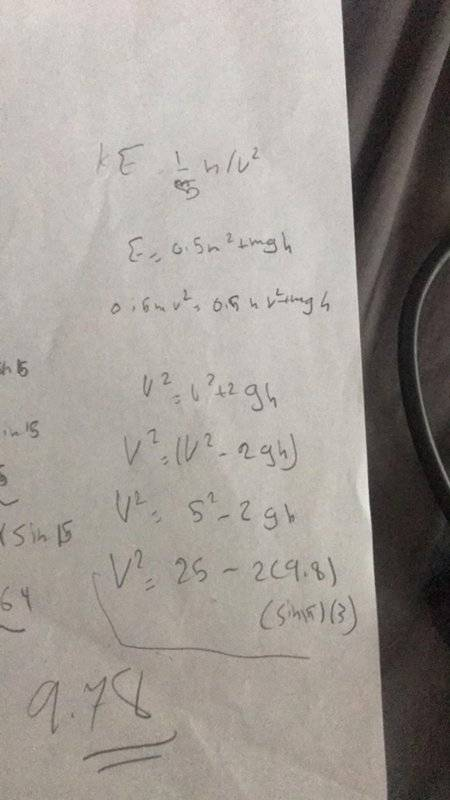# Your help is appreciated -- Skateboarder on a ramp

meso
A skateboarder coasts at a constant velocity toward a ramp with a 15° angle, preparing for the big trick jump. If the frictionless ramp is 3m long, and his initial velocity at the base of the ramp is 5 m/s, calculate
a) his final speed at the end of the ramp

Mentor
A skateboarder coasts at a constant velocity toward a ramp with a 15° angle, preparing for the big trick jump. If the frictionless ramp is 3m long, and his initial velocity at the base of the ramp is 5 m/s, calculate
a) his final speed at the end of the ramp

Welcome to the PF.I've moved your thread to the Homework Help forums, where all schoolwork problems should be posted.

Also, please list the Relevant Equations that you think apply to this problem.

Finally, the problem statement seems incomplete. Is the skateboarder going UP the ramp, or DOWN the ramp? If you are going to use energy considerations to figure out the change in velocity, you need to know the total distance up or down that they travel, and I don't see enough information in your statement to figure that out.

Is there a diagram that goes with this question? You can use the "Attach files" button to add attachments (PDF, JPEG, etc.) to your reply...

mesomeso
Welcome to the PF.I've moved your thread to the Homework Help forums, where all schoolwork problems should be posted.

Also, please list the Relevant Equations that you think apply to this problem.

Finally, the problem statement seems incomplete. Is the skateboarder going UP the ramp or DOWN the ramp? If you are going to use energy considerations to figure out the change in velocity, you need to know the total distance up or down that they travel, and I don't see enough information in your statement to figure that out.

Is there a diagram that goes with this question? You can use the "Attach files" button to add attachments (PDF, JPEG, etc.) to your reply...
Thank you so much! My teacher posted this as an assignment question, no further information provided. What I know is that I need to use the gravity force components. This equation might be useful v2^2=v1^2+2ad

Mentor
The ramp is up or down? Will the skateboarder be going faster or slower at the end of the ramp? Normally the ramp would be down to increase speed, but the word "base" of the ramp for the start implies that the ramp is upward, so the rider would be slowing down going up the ramp, right?

If the ramp goes down, you only need to figure out the vertical drop and use the change in potential energy (PE) between the top and bottom of the ramp to figure out how much kinetic energy (KE) the skateboarder gains, and translate that into the increase in velocity...

In your hand-written workings here you have assumed an upward sloping ramp:$$v_f^2 = v_i^2 + 2gh$$ but since we are adopting an upward positive sign convention and since the acceleration of gravity is negative, this is immediately rewritten as:$$v_f^2 = v_i^2 - 2gh$$It is not really kosher to switch sign conventions in midstream like this, but I think I've understood the intent.
You next substitute in the known initial velocity 5 for ##v_i## to obtain:$$v_f^2=5^2-2gh$$ and you substitute in 9.8 for g, and ##3 \sin 15## for h yielding$$v_f^2 = 5^2 - 2(9.8)(3 \sin 15)$$ You evaluate the right hand side obtaining $$9.78$$ but you've missed something important. The left hand side was not ##v_f##.
•